﻿ 多路温度远传监测仪原理图、设计思路和参数计算方法-昌晖仪表网

# 多路温度远传监测仪原理图、设计思路和参数计算方法

2020/1/11 0:45:56 人评论 次浏览 分类：二次仪表  文章地址：http://yunrun.com.cn/tech/2872.html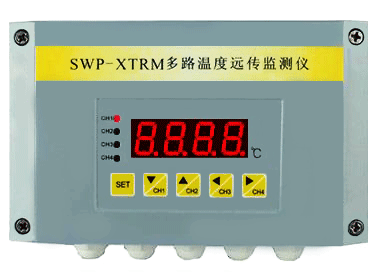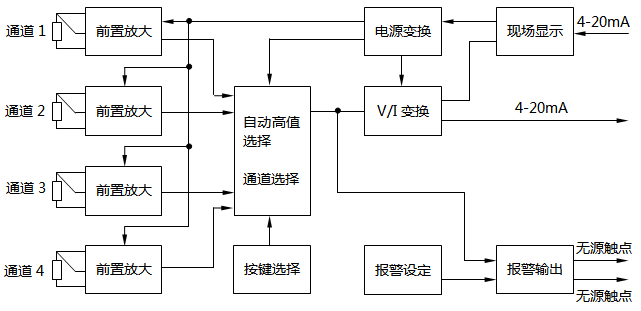1、电源电路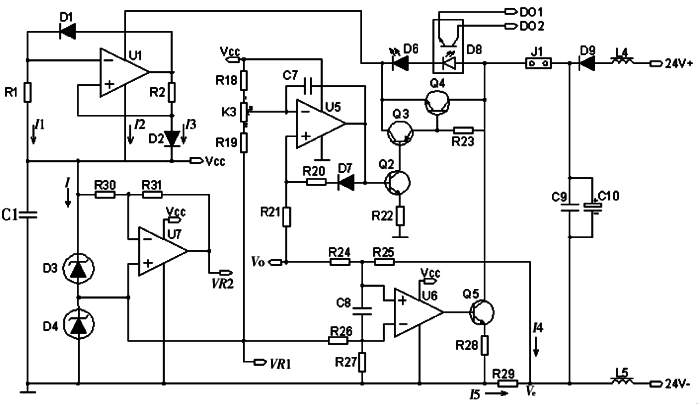2、铂电阻接口电路

Rt=R0[1+At+Bt2+C(t-100)t3]表明Rt=f(t)是非线性函数，曲线呈上凸特性。以Pt100为例，设步长为0.1℃，从-200℃开始，逐点代入式I=I1+I2+I3计算，得到铂热电阻传感器的非线性特性(图3)。结果表明：量程范围内最大非线性误差达到4.5%，必须采取线性化措施。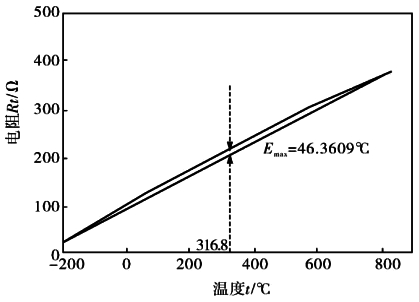②导线电阻补偿电路

③线性放大电路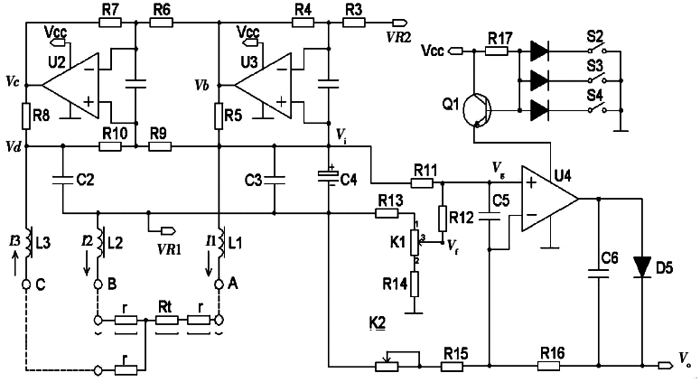f=-[(K113+R13)×VR1]/(R13+K1+R14)；由叠加定理得Vg(相对VR1)为：Vg=(Vi×R12)/(R11+R12)+(Vf×R11)/(R11+R12)；由U4工作原理可得：Vo=[(R11×Vf)/(R11+R12)+(R12×Vi)/(R11+R12)]×[1+R16/(K2+R15)]，设K=1+R16/(K2+R15)，可得：Vo=[(R11×Vf)/(R11+R12)+(R12×Vi)/(R11+R12)]×K=[(R1×2K)/(R11+R12)]×Vi+[(R11×Vf)/(R11+R12)]×K，由此可知，电压Vo和电压Vi成线性关系，可由电位器K1调整截距，由电位器K2调整斜率。

④通道选择电路

⑤V/I转换及显示电路

V/I转换电路如图2右下部分所示。它将电压Vo经过V/I电路变成电流Io(4-20mA)输出。输出电流Io等于电流I4和I5之和，因为I4非常微小，所以输出电流Io的大小只与R29和Vo有关。设R24、R25、R26和R27均等于500kΩ，Ve为相对VR1的电压，根据运放U6的工作原理可得：Vo+V2=0-VR1和Io=I5=(-VR1-Ve)/R29=Vo/R29

⑥报警电路

1、电源电路
①工作电流计算

②参考电压计算

Vcc=VD1+VD2=1.25+2.50=3.75V，而运放的最小正常工作电压为2.50V，参考电压满足设计要求。VR1=VD1=1.25V。取R31=56kΩ，R30=200kΩ，由式VR2=VR1-[(Vcc-VR1)×R31]/R30得VR2=1.25-[(3.75-1.25)×56]/200=0.55V。

2、铂电阻接口电路参数计算

3、信号放大电路参数计算

①输出电压Vo范围值的确定
Rt的取值范围为100.00~157.33Ω，将这个范围值与R5、VR1和VR2的值代入式Vi=[(VR1-VR2)×Rt]/(R5-Rt)=[(VR1-VR2)/R5]×[Rt/(1-Rt/R5)]中，求得Vi的取值范围为27.5~44.2mV。

②Vo的斜率和截距的确定

Vi的范围为27.5~44.2mV，且Vo的范围为100~500mV，则Vi和Vo满足下式：Vo=23.95×Vi-0.76

④报警电路参数计算

 与在线工程师QQ交流 到论坛与网友交流 向昌晖仪表网投稿 免费获得2000元电子书

客户姓名：